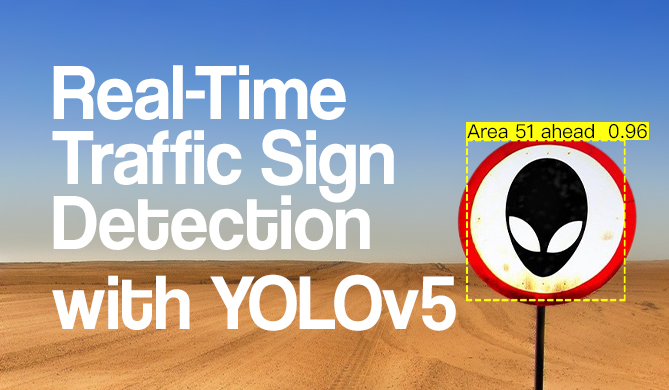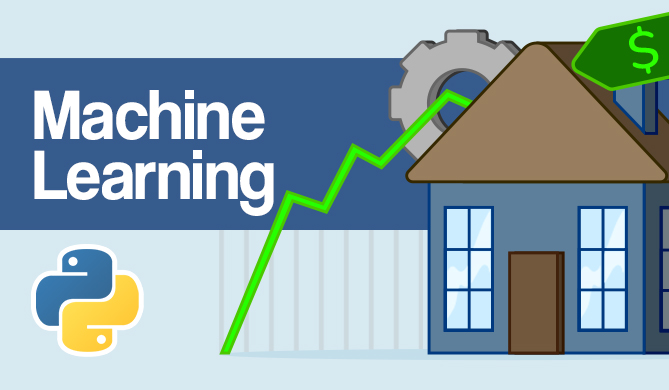End-to-End Random Forest Regression Pipeline with Scikit-Learn

# End-to-End Random Forest Regression Pipeline with Scikit-LearnRegression is a technique in statistics and machine learning, in which the value of an independent variable is predicted by its relationship with other variables.

Frameworks like Scikit-Learn make it easier than ever to perform regression with a wide variety of models - one of the strongest ones being built on the Random Forest algorithm.

If you'd like to read an in-depth guide to Random Forests, read our "Random Forest Algorithm with Python and Scikit-Learn"!

Using Scikit-Learn pipelines, you can build an end-to-end pipeline, load a dataset, perform feature scaling and and supply the data into a regression model in as little as 4 lines of code:

from sklearn import datasets
from sklearn.model_selection import train_test_split
from sklearn.preprocessing import MinMaxScaler
from sklearn.ensemble import RandomForestRegressor
from sklearn.pipeline import Pipeline

X, y = datasets.fetch_california_housing(return_X_y=True)
X_train, X_test, y_train, y_test = train_test_split(X, y)

pipeline = Pipeline([('scaler', MinMaxScaler()), ('regressor', RandomForestRegressor())])
pipeline.fit(X_train, y_train)

r2 = pipeline.score(X_test, y_test)
print(f"RFR: {r2}") # RFR: 0.811299774049597


Alternatively, you can separate the steps outside of the pipeline, which is a bit more verbose, yet more flexible:

X, y = datasets.fetch_california_housing(return_X_y=True)
X_train, X_test, y_train, y_test = train_test_split(X, y)

scaler = MinMaxScaler()
X_train_scaled = scaler.fit_transform(X_train)
X_test_scaled = scaler.transform(X_test)

rfr = ensemble.RandomForestRegressor().fit(X_train_scaled, y_train)

r2 = rfr.score(X_test_scaled, y_test)
print(f"RFR: {r2}") # RFR: 0.8039270852048439

Last Updated: July 1st, 2022

### You might also like...

David LandupAuthor

Entrepreneur, Software and Machine Learning Engineer, with a deep fascination towards the application of Computation and Deep Learning in Life Sciences (Bioinformatics, Drug Discovery, Genomics), Neuroscience (Computational Neuroscience), robotics and BCIs.

Great passion for accessible education and promotion of reason, science, humanism, and progress.Project

### Real-Time Road Sign Detection with YOLOv5

# python# machine learning# computer vision# pytorch

If you drive - there's a chance you enjoy cruising down the road. A responsible driver pays attention to the road signs, and adjusts their...David Landup
DetailsProject

### Hands-On House Price Prediction - Machine Learning in Python

# python# machine learning# scikit-learn# tensorflow

If you've gone through the experience of moving to a new house or apartment - you probably remember the stressful experience of choosing a property,...Details